# 『前端BUG』—— toFixed四舍五入的不准确性

## 缘由

chrome：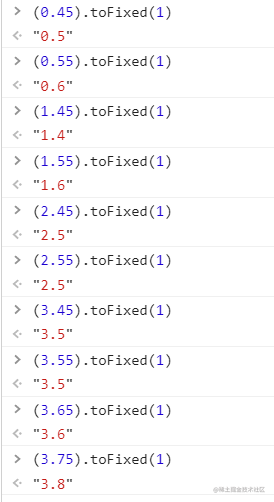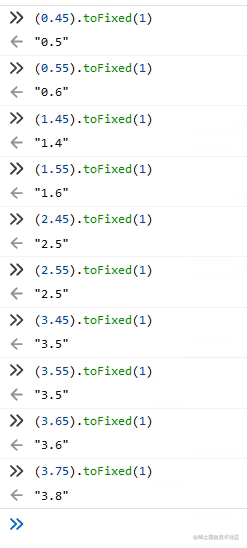IE: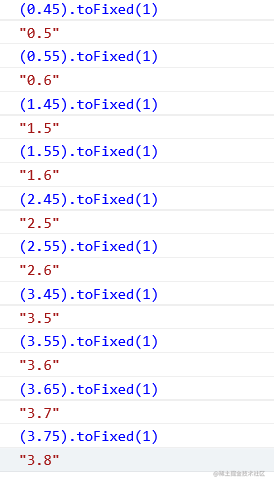toFixed()在chrome、火狐上也并不是网上所说的用银行家舍入法来进行四舍五入的。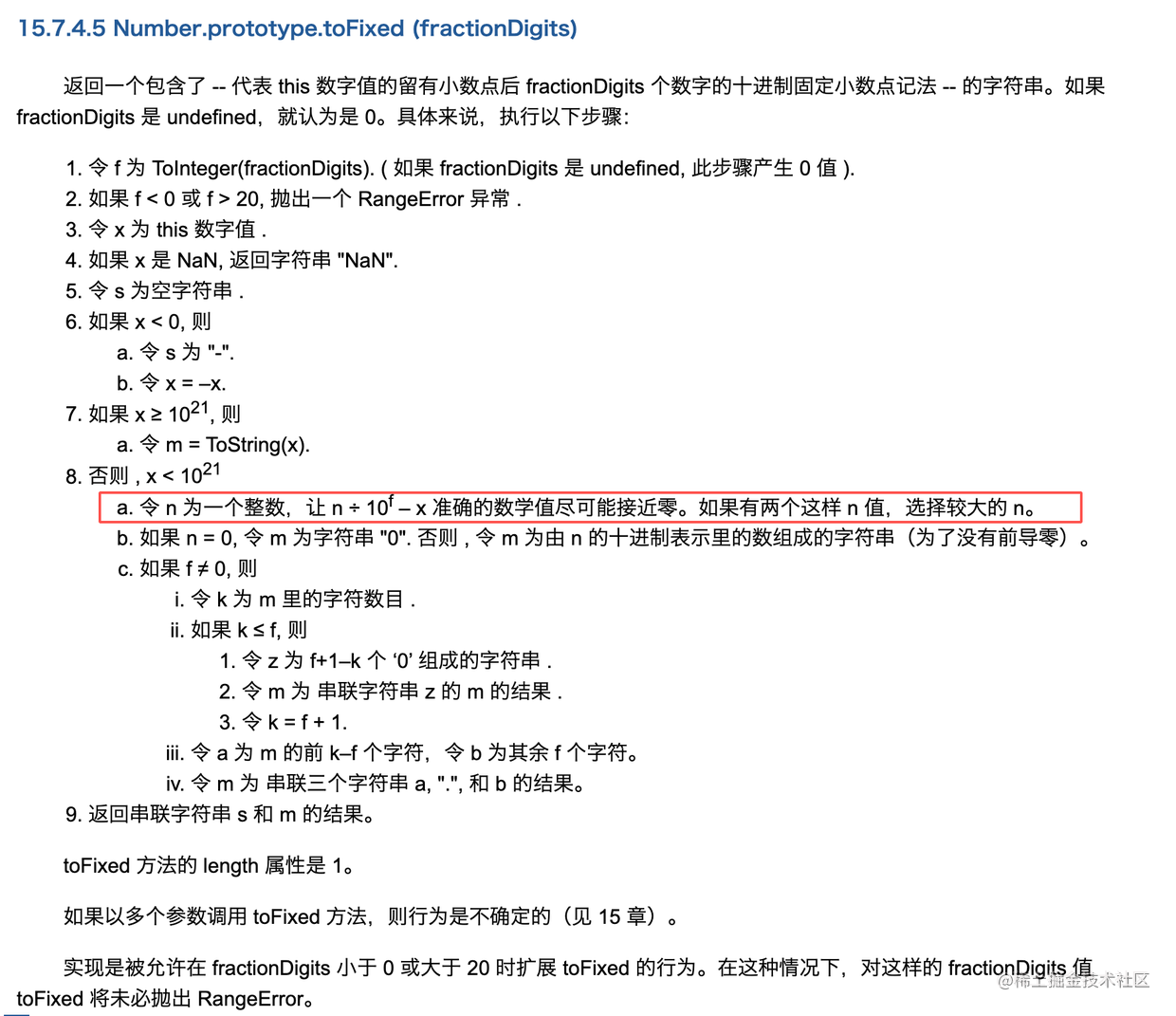x为2.55，小于$10^{21}$，f为1，要使$n\div10^f-x$准确的数学值尽可能接近零，取n为25和n为26，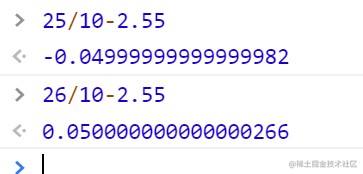## 解决

function toFixed(number, m) {
if (typeof number !== 'number') {
throw new Error("number不是数字");
}
let result = Math.round(Math.pow(10, m) * number) / Math.pow(10, m);
result = String(result);
if (result.indexOf(".") == -1) {
if(m != 0){
result += ".";
result += new Array(m + 1).join('0');
}
} else {
let arr = result.split('.');
if (arr.length < m) {
arr += new Array(m - arr.length + 1).join('0')
}
result = arr.join('.')
}
return result
}